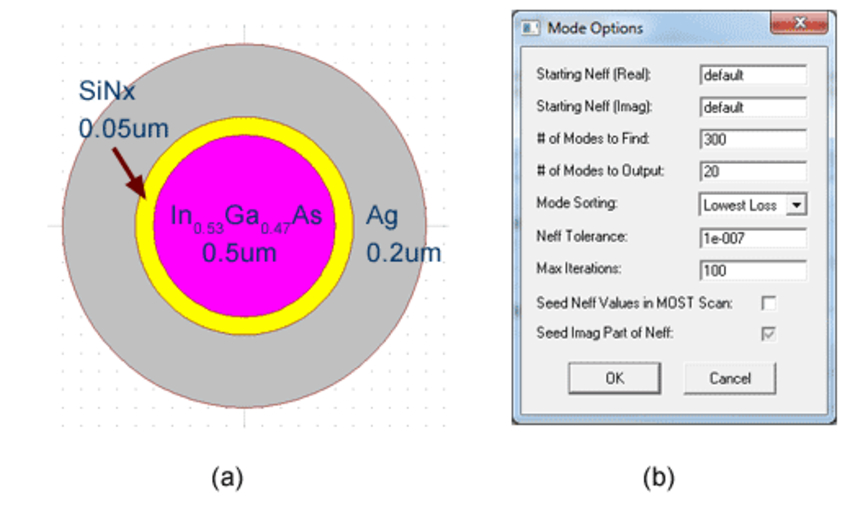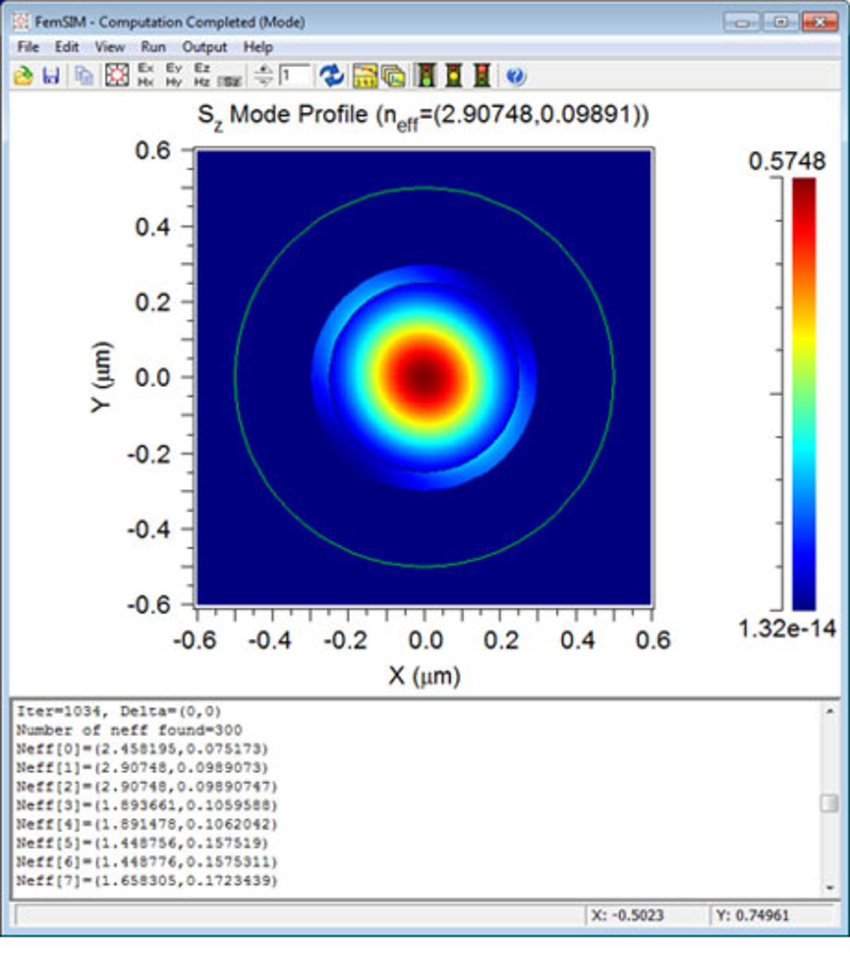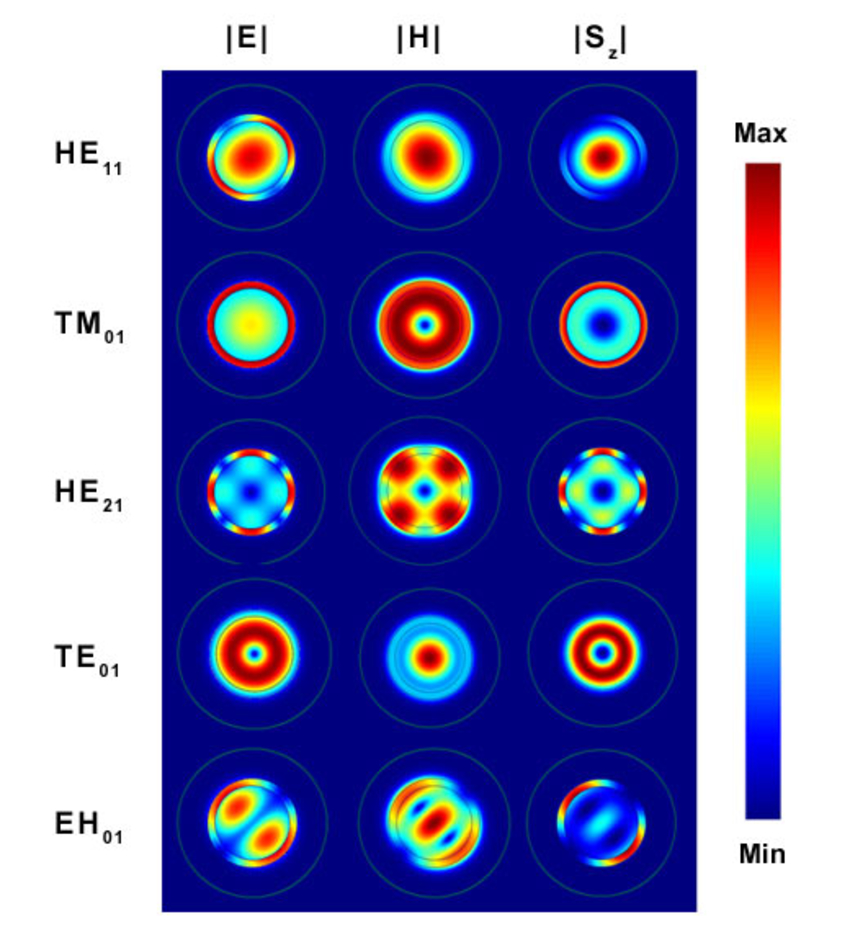# Performing Multimode Calculations of a Metal Clad Waveguide Using FemSIM

Finite Element Mode solver, FemSIM, is a powerful tool to calculate waveguide modes with a full vector solution, and will simultaneously solve for guided, leaky, and radiation modes. When designing a structure that supports a large number of leaky or radiation modes, it is common to want to solve only for the guided modes. To do this in FemSIM, we can solve for a large number of modes and sort them by lowest loss. Sorting by lowest loss will bring guided modes to the top of the list, since they typically have a lower loss than leaky/radiation modes. Additionally, FemSIM can output a subset of the calculated modes so it is not necessary to save all the computed leaky/radiation modes.

This article will demonstrate the use of FemSIM to compute the modes in a metal-clad circular waveguide used in a sub-wavelength metal cladded disc laser. Mode analysis will help to determine the optimum use of the gain materials (1). The design files referenced in this article are available on our Customer Support Portal.

Figure 1(a) shows the cross section of the metal clad waveguide. Figure 1(b) shows the Mode Options dialog box for this waveguide. In order to calculate only the guided modes in this simulation, we ask FemSIM to calculate 300 modes, sort the calculated modes by lowest loss, and then output the 20 modes with the lowest loss. Additionally, note that the H-field formulation was used for this simulation due to the discontinuity of the E field at the metal interface. At the end of the simulation, FemSIM will output all six field components (E,H) of the computed modes as well as the Poynting vector in the Z direction (Sz). Figure 2 shows Sz for one of the calculated modes.Figure 1: (a) Cross Section of Metal Clad Waveguide with materials and dimensions;
(b) Mode Option Settings for multimode FemSIM calculationFigure 2: FemSIM simulation display window after simulation is completed

Sometimes users want to view the |E| and |H| distributions for the calculated modes. We can use the mathmat utility to obtain this result easily. The mathmat commands that can be used to obtain |E| and |H| are:

mathmat  “sqrt(__a^2+__b^2+__c^2)” Mode_ex.m00 Mode_ey.m00 Mode_ez.m00 > Mode_E.m00
mathmat  “sqrt(__a^2+__b^2+__c^2)” Mode_hx.m00 Mode_hy.m00 Mode_hz.m00 > Mode_H.m00

where ‘Mode’ is the Output Prefix used for the simulation. Figure 3 shows the |E|, |H|, and Sz distributions of five modes for this waveguide.Figure 3: Five lowest order mode patterns (|E|, |H|, and Sz) are plotted correspondingly. Power nodes at the waveguide center are observed in TM01, HE21, and TE01 modes.

Please contact our technical support team at photonics_support@synopsys.com for more information.

References

1. C. Lu and S. Chuang, "A surface-emitting 3D metal-nanocavity laser: proposal and theory," Opt. Express 19, 13225-13244 (2011).